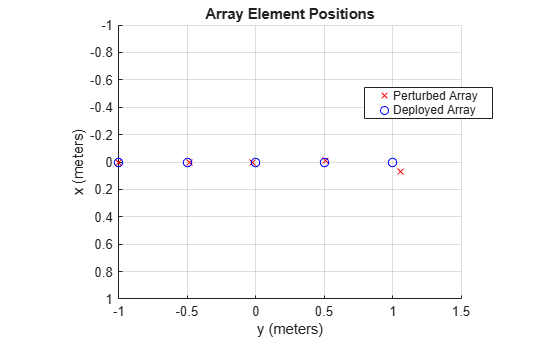# Using Self Calibration to Accommodate Array Uncertainties

This example shows a self calibration procedure based on a constrained optimization process. Sources of opportunity are exploited to simultaneously estimate array shape uncertainties and source directions.

This example requires Optimization Toolbox™.

### Introduction

In theory, one can design a perfect uniform linear array (ULA) to perform all sorts of processing such as beamforming or direction of arrival estimation. Typically this array will be calibrated in a controlled environment before being deployed. However, uncertainties may arise in the system during operation indicating that the array needs recalibrating. For instance, environmental effects may cause array element positions to become perturbed, introducing array shape uncertainties. The presence of these uncertainties causes rapid degradation in the detection, resolution and estimation performance of array processing algorithms. It is therefore critical to remove these array uncertainties as soon as possible.

There are many array calibration algorithms. This example focuses on one class of them, self calibration (also called auto-calibration), where uncertainties are estimated jointly with the positions of a number of external sources at unknown locations . Unlike pilot calibration, this allows an array to be re-calibrated in a less known environment. However, in general, this results in a small number of signal observations with a large number of unknowns. There are a number of approaches to solving this problem as described in . One is to construct and optimize against a cost function. These cost functions tend to be highly non-linear and contain local minima. In this example, a cost function based on the Multiple Signal Classification (MUSIC) algorithm  is formed and solved as an fmincon optimization problem using Optimization Toolbox (TM). In the literature, many other combinations also exist .

### A Perfect Array

Consider first a 5-element ULA operating with half wavelength spacing is deployed. In such an array, the element positions can be readily computed.

```N = 5; designed_pos = [zeros(1,N);-(N-1)/2:(N-1)/2;zeros(1,N)]*0.5;```

### A Not So Perfect Array

Next, assume the array is perturbed whilst in operation and so undergoes array shape uncertainties in the x and y dimensions. In order to fix the global axes, assume that the first sensor and the direction to the second sensor is known as prescribed in .

```rng default pos_std = 0.02; perturbed_pos = designed_pos + pos_std*[randn(2,N);zeros(1,N)]; perturbed_pos(:,1) = designed_pos(:,1); % The reference sensor has no % uncertainties perturbed_pos(1,2) = designed_pos(1,2); % The x axis is fixed down by % assuming the x-location of % another sensor is known```

### Visualize the Array Imperfections

The figure below shows the difference between the deployed and the perturbed array.

```helperCompareArrayProperties('Position',perturbed_pos,designed_pos,... {'Perturbed Array','Deployed Array'}); view(90,90);```The previous section shows the difference between the deployed array and an array which has undergone perturbations while in operation. If one blindly uses the processing designed for the deployed array, the performance of the array reduces. For example, consider a beamscan estimator is used to estimate the directions of 3 unknown sources at -20, 40 and 85 degrees azimuth.

```% Generate 100K samples with 30dB SNR ncov = db2pow(-30); Nsamp = 1e5; % Number of snapshots (samples) incoming_az = [-20,40,85]; % Unknown source locations to be estimated M = length(incoming_az); [x_pert,~,Rxx] = sensorsig(perturbed_pos,Nsamp,incoming_az,ncov); % Estimate the directions of the sources ula = phased.ULA('NumElements',N); spatialspectrum = phased.BeamscanEstimator('SensorArray',ula,... 'DOAOutputPort',true,'NumSignals',M); [y,estimated_az] = spatialspectrum(x_pert); incoming_az```
```incoming_az = 1×3 -20 40 85 ```
`estimated_az`
```estimated_az = 1×3 -19 48 75 ```

These uncertainties degrade the array performance. Self calibration can allow the array to be re-calibrated using sources of opportunity, without needing to know their locations.

### Self Calibration

A number of self calibration approaches are based on optimizing a cost function to jointly estimate unknown array and source parameters (such as array sensor and source locations). The cost function and optimization algorithm must be carefully chosen to encourage a global solution to be reached as easily and quickly as possible. In addition, parameters associated with the optimization algorithm must be tuned for the given scenario. A number of combinations of cost function and optimization algorithm exist in the literature. For this example scenario, a MUSIC cost function  is chosen alongside an fmincon optimization algorithm. As the scenario changes, it may be appropriate to adapt the approach used depending upon the robustness of the calibration algorithm. For instance, in this example, the performance of the calibration algorithm drops as sources move away from end-fire or the number of array elements increase. The initial estimates of the source locations estimated previously are used as the initialization criterion of the optimization procedure.

```fun = @(x_in)helperMUSICIteration(x_in,Rxx,designed_pos); nvars = 2*N - 3 + M; % Assuming 2D uncertainties x0 = [0.1*randn(1,nvars-M),estimated_az]; % Initial value locTol = 0.1; % Location tolerance angTol = 20; % Angle tolerance lb = [-locTol*ones(nvars-M,1);estimated_az.'-angTol]; % lower bound ub = [locTol*ones(nvars-M,1);estimated_az.'+angTol]; % upper bound options = optimoptions('fmincon','TolCon',1e-6,'DerivativeCheck','on',... 'Display','off'); [x,fval,exitflag] = fmincon(fun,x0,[],[],[],[],lb,ub,[],options); % Parse the final result [~,perturbed_pos_est,postcal_estimated_az]=helperMUSICIteration(... x,Rxx,designed_pos);```
```helperCompareArrayProperties('Position',perturbed_pos,perturbed_pos_est,... {'Perturbed Array','Calibrated Array'}); view(90,90);``````polarplot(deg2rad(incoming_az),[1 1 1],'s',... deg2rad(postcal_estimated_az(1,:)),[1 1 1],'+',... deg2rad(estimated_az),[1 1 1],'o','LineWidth',2,'MarkerSize',10) legend('True directions','DOA after calibration',... 'DOA before calibration','Location',[0.01 0.02 0.3 0.13]) rlim([0 1.3])```By performing this calibration process the accuracy of the source estimation has improved significantly. In addition, the positions of the perturbed sensors have also been estimated which can be used as the new array geometry in the future.

### Summary

This example shows how array shape uncertainties can impact the ability to estimate the direction of arrival of unknown sources. The example also illustrates how self calibration can be used to overcome the effects of these perturbations and estimate these uncertainties simultaneously.

### References

Van Trees, H. Optimum Array Processing. New York: Wiley-Interscience, 2002.

 E Tuncer and B Friedlander. Classical and Modern Direction-of-Arrival Estimation. Elsevier, 2009.

 Schmidt, R. O. "Multiple Emitter Location and Signal Parameter Estimation." IEEE Transactions on Antennas and Propagation. Vol. AP-34, March, 1986, pp. 276-280.

 Y. Rockah and P. M. Schultheiss. Array shape calibration using sources in unknown locations- Part I: Farfield sources. IEEE Trans. ASSP, 35:286-299, 1987.